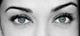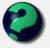# Math Is Fun Forum

Discussion about math, puzzles, games and fun.   Useful symbols: ÷ × ½ √ ∞ ≠ ≤ ≥ ≈ ⇒ ± ∈ Δ θ ∴ ∑ ∫  π  -¹ ² ³ °

You are not logged in.

## #1 2007-02-20 12:52:12

unique
MemberRegistered: 2006-10-04
Posts: 419

### HelpDesi
Raat Key Rani !

Offline

## #2 2007-02-20 13:27:11

mathsyperson
ModeratorRegistered: 2005-06-22
Posts: 4,900

### Re: Help

I'm assuming the text is meant to say

This figure is the base of a cone that is 8 inches tall.
What is the volume of the cone? Dimensions are in inches.

It's a curious property of cones that no matter how irregularly shaped its base is, its volume can always be worked out by (basearea x height)/3.

So if we can work out the area of that base, then the rest is easy.
The base is composed of a right-angled triangle and a sector of a circle.

Triangle: (8*12)/2 = 48.
Sector: π*8² * (50/360) = 80π/9.

Therefore, the total area of the base is 48 + 80π/9, which is ~ 75.9 square inches.

From here, we just need to multiply by the height and divide by 3, which gives a final area of:
(8/3)(48 + 80π/9) cubic inches if you're being exact, or ~202 cubic inches if you're approximating.

Why did the vector cross the road?
It wanted to be normal.

Offline